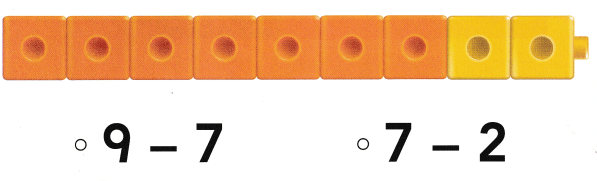# Texas Go Math Kindergarten Lesson 10.6 Answer Key Decompose 8 and 9

Refer to our Texas Go Math Kindergarten Answer Key Pdf to score good marks in the exams. Test yourself by practicing the problems from Texas Go Math Kindergarten Lesson 10.6 Answer Key Decompose 8 and 9.

## Texas Go Math Kindergarten Lesson 10.6 Answer Key Decompose 8 and 9

Essential Question
How do you take away numbers from 8 and 9?

Explore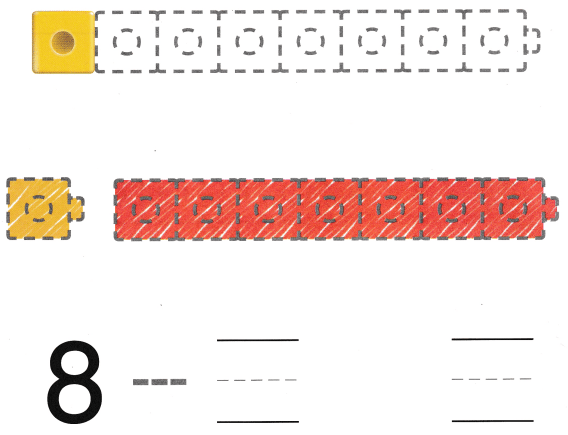Explanation:
One cube is yellow and the rest are red.
total there are 8 cubes
8 – 1 = 7
so, 7 are red.

Directions
Model an eight-cube train. One cube is yellow and the rest are red. Take apart the train to model the set taken apart. Draw and color the cube trains. Trace the symbol and write the numbers to show 8 taken apart.

Share and Show

Question 1.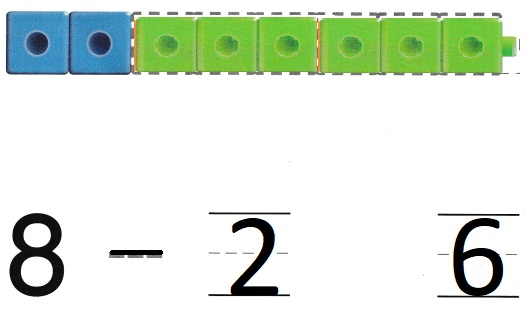Explanation:
Two cubes are blue and the rest are green.
There are 8 cubes in total.
8 – 2 = 6
so, there are 6 green cubes.

Question 2.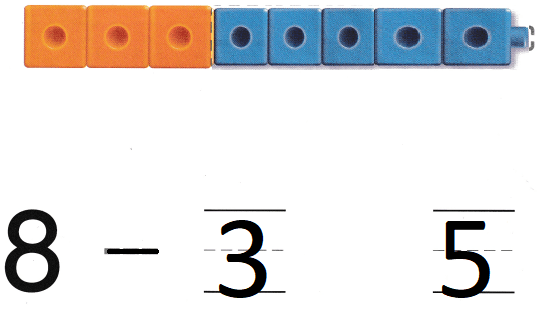Explanation:
Three cubes are orange and the rest are blue.
total there are 8 cubes
8 – 3 = 5
so, there are 5 cubes in all.

Directions
1.
Model an eight-cube train. Two cubes are blue and the rest are green. Take apart the train to model the set taken apart. Draw and color the cube trains. Trace the symbol and write the numbers to show 8 taken apart. 2. Model an eight-cube train. Three cubes are orange and the rest are blue. Take apart the train to model the set taken apart. Draw and color the cube trains. Trace the symbol and write the numbers to show 8 taken apart.

Question 3.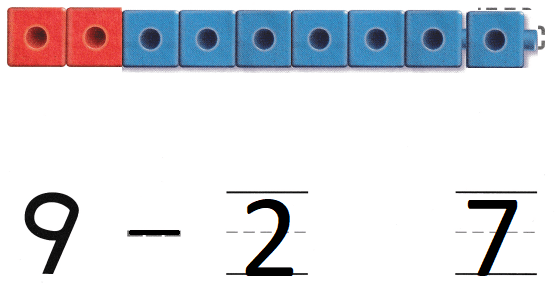Explanation:
Two cubes are red and the rest are blue.
total there are 9 cubes
9 – 2 = 7
so, 7 cubes are blue

Question 4.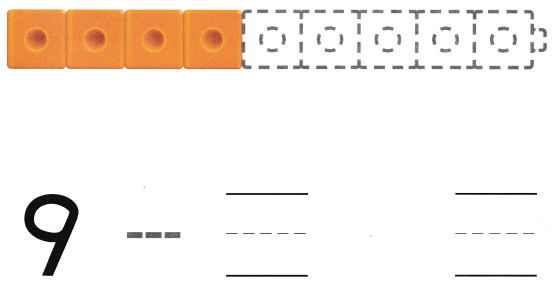Explanation:
Four cubes are orange and the rest are green.
Total there are 9 cubes
9 – 4 = 5
so, 5 cubes are green.

Directions
3. Model a nine-cube train. Two cubes are red and the rest are blue. Take apart the train to model the set taken apart. Draw and color the cube trains. Trace the symbol and write the numbers to show 9 taken apart. 4. Model a nine-cube train. Four cubes are orange and the rest are green. Take apart the train to model the set taken apart. Draw and color the cube trains. Trace the symbol and write the numbers to show 9 taken apart.

Home Activity

• Find 8 or 9 small household objects. Line them up and have your child take apart two sets. Have your child tell you how many objects are in each set.

Problem Solving

Question 5.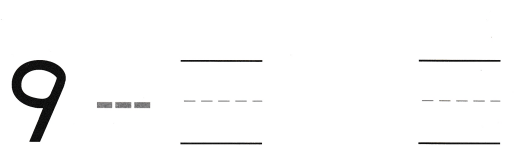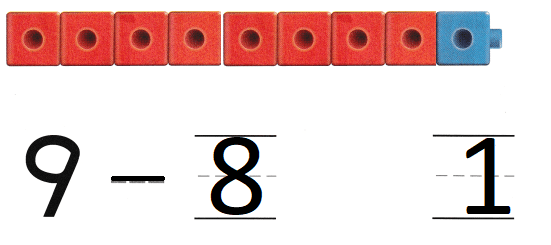Explanation:
Michelle has 9 cubes. 8 cubes are red.
The rest of the cubes are blue.
9 – 8 = 1
so, 1 cube is blue.

Question 6.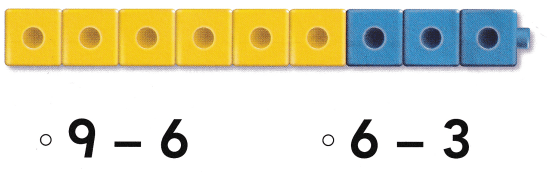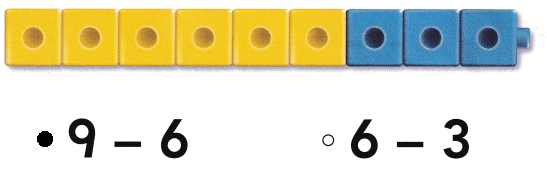Explanation:
There is a nine cube train
6 cubes are yellow
so, 9 – 6 = 3
the rest three are blue

Directions
5. Michelle has 9 cubes. 8 cubes are red. The rest of the cubes are blue. How many cubes are blue? Draw the cubes. Trace the symbol and write the numbers. 6. Choose the correct answer. Which does the cube train show?

### Texas Go Math Kindergarten Lesson 10.6 Homework and Practice

Question 1.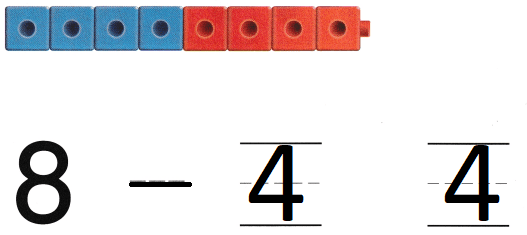Explanation:
Four cubes are blue and the rest are red.
Total number of cubes are 8
8 – 4 = 4
so, there are 4 red cubes.

Question 2.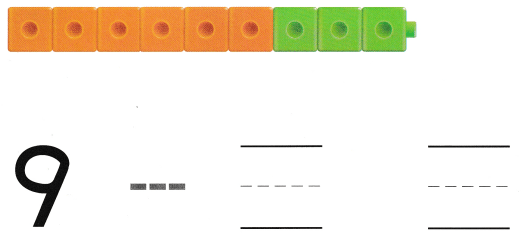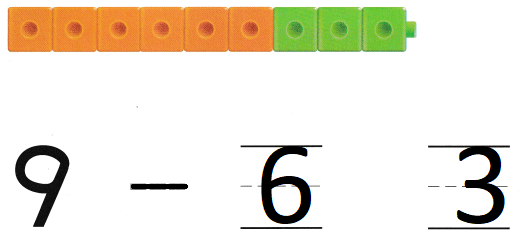Explanation:
Six cubes are orange and the rest are green.
Total number of cubes are 9
9 – 6 = 3
so, there are 3 green cubes.

Directions
1. Look at the eight-cube train. Four cubes are blue and the rest are red. Take apart the train by drawing to model the set taken apart. Color the trains. Trace and write to show 8 taken apart. 2. Look at the nine-cube train. Six cubes are orange and the rest are green. Take apart the train by drawing to model the set taken apart. Color the trains, Trace and write to show 9 taken apart.

Texas Test Prep

Lesson Check

Question 3.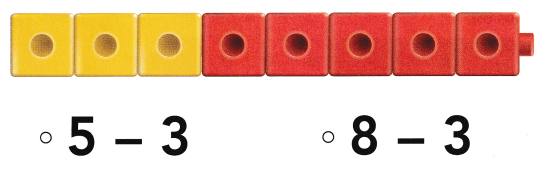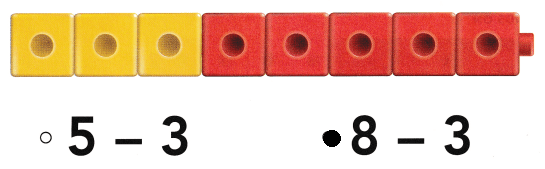Explanation:
The given cube train has 8 cubes
3 are yellow and the rest are red
so, 8 – 3 = 5
5 are red cubes.

Question 4.